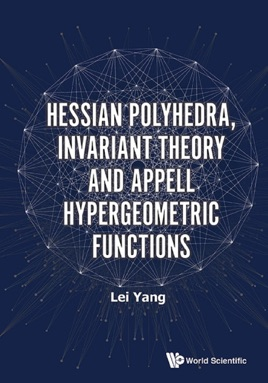• \$114.99

## Publisher Description

Our book gives the complex counterpart of Klein's classic book on the icosahedron. We show that the following four apparently disjoint theories: the symmetries of the Hessian polyhedra (geometry), the resolution of some system of algebraic equations (algebra), the system of partial differential equations of Appell hypergeometric functions (analysis) and the modular equation of Picard modular functions (arithmetic) are in fact dominated by the structure of a single object, the Hessian group 𝔊′216. It provides another beautiful example on the fundamental unity of mathematics.

Contents:Geometry and Arithmetic Associated with Appell Hypergeometric Functions:IntroductionFour Derivatives and Their PropertiesNonlinear Partial Differential Equations and Modular Functions Associated to U(2, 1)η-Functions Associated to U(2, 1) and Picard CurvesTransform Problems and Modular Equations Associated to Algebraic SurfacesTriangular S-Functions Associated to U(2, 1) and Picard CurvesFour Derivatives and Nonlinear Evolution EquationsHessian Polyhedra, Invariant Theory and Appell Hypergeometric Functions:IntroductionThe Reciprocity Law About the Appell Hypergeometric FunctionsThe Algebraic Solutions of Appell Hypergeometric Partial Differential EquationsThe Hessian Polyhedral EquationsInvariant Theory for the System of Algebraic EquationsTernary Cubic Forms Associated to Hessian PolyhedraSome Rational Invariants on ℂℙ2Galois Representations Arising from Twenty-Seven Lines on a Cubic Surface and the Arithmetic Associated with Hessian Polyhedra:IntroductionHessian Polyhedra and Cubic Forms Associated to G25,920Hessian Polyhedra and Picard Modular FormsHessian Polyhedra and Galois Representations Associated with Cubic SurfacesHessian Polyhedra and the Arithmetic of Rigid Calabi-Yau Threefolds